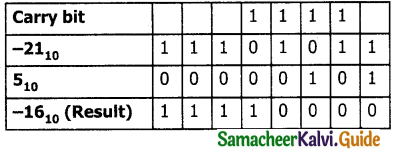Tamilnadu State Board New Syllabus Samacheer Kalvi 11th Computer Applications Guide Pdf Chapter 2 Number Systems Text Book Back Questions and Answers, Notes.

## Tamilnadu Samacheer Kalvi 11th Computer Applications Solutions Chapter 2 Number Systems

### 11th Computer Applications Guide Number Systems Text Book Questions and Answers

Part I

Question 1.
Which refers to the number of bits processed by a computer’s CPU?
a) Byte
b) Nibble
c) Word length
d) Bit
c) Word length

Question 2.
How many bytes does 1 KiloByte contain?
a) 1000
b) 8
c) 4
d) 1024
d) 1024Question 3.
Expansion for ASCII _________.
a) American School Code for Information Interchange
b) American Standard Code for Information Interchange
c) All Standard Code for Information Interchange
d) American Society Code for Information Interchange
b) American Standard Code for Information Interchange

Question 4.
2^50 is referred as _________.
a) Kilo
b) Tera
c) Peta
d) Zetta
c) Peta

Question 5.
How many characters can be handled in Binary Coded Decima System?
a) 64
b) 255
c) 256
d) 128
a) 64

Question 6.
For 11012 what is the Hexadecimal equivalent?
a) F
b) E
c) D
d) B
c) D

Question 7.
What is the 1’s complement of 00100110?
a) 00100110
b) 11011001
c) 11010001
d) 00101001
b) 11011001Question 8.
Which amongst this is not an Octal number?
a) 645
b) 234
c) 876
d) 123
c) 876

Part – II

Question 1.
What is data?
The data is a fact about people, places or some object. In a program, a value assigned to a variable is called a data.

Question 2.
Write the 1’s complement procedure.
The steps to be followed to find l’s complement of a number:

• Step 1: Convert given Decimal number into Binary
• Step 2 : Check if the binary number contains 8 bits , if less add 0 at the left most bit, to make it as 8 bits.
• Step 3 : Invert all bits(i.e. change 1 as 0 and 0 as 1)
Example : Find 1’s complement for (-24)10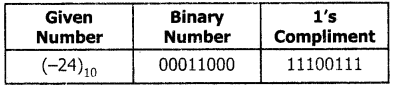Question 3.
Convert (46)10 into Binary number.Question 4.
We cannot find 1’s complement for (28)10. State reason.
Complement is only for negative number. (28)10 is a positive number . So we can’t find 1’s complement.Question 5.
List the encoding systems for characters in memory.
There are several encoding systems used for computer. They are

1. BCD – Binary Coded Decimal
2. EBCDIC – Extended Binary Coded Decimal Interchange Code
3. ASCII – American Standard Code for Information Interchange
4. Unicode
5. ISCII – Indian Standard Code for Information Interchange

Part – III

Question 1.
What is radix of a number system? Give
example.
Each number system is uniquely identified by its base value or radix. Radix or base ¡s the count of number of digits in each number system. Radix or base is the general idea behind positional numbering system.

Example:Question 2.
Write note on binary number system.
There are only two digits in the Binary system, namely, 0 and 1. The numbers in the binary system are represented to the base 2 and the positional multipliers are the powers of 2.

The left most bit in the binary number is called as the Most Significant Bit (MSB) and it has the largest positional weight. The right most bit is the Least Significant Bit (LSB) and has the smallest positional weight.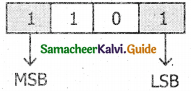Question 3.
Convert (150)10 into Binary, then convert that Binary number to Octal.
Decimal to Binary conversion 150(150)10 = (10010110)2
Binary to octal conversion
LSB to MSB divide the number into three digit binary and write the equivalent octal digit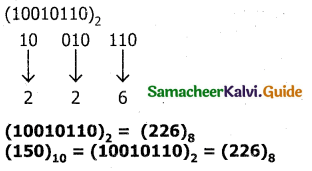Question 4.
Write short note on ISCII.
Indian Standard Code for Information Interchange (ISCII):
ISCII is the system of handling the character of Indian local languages. This is an 8-bit coding system. Therefore it can handle 256 (28) characters.

This system is formulated by the department of Electronics in India in the year 1986-88 and recognized by Bureau of Indian Standards (BIS). Now this coding system is integrated with Unicode.

Question 5.
Add : a) -2210 + 1510
b) 2010 + 2510.
a) -2210 + 1510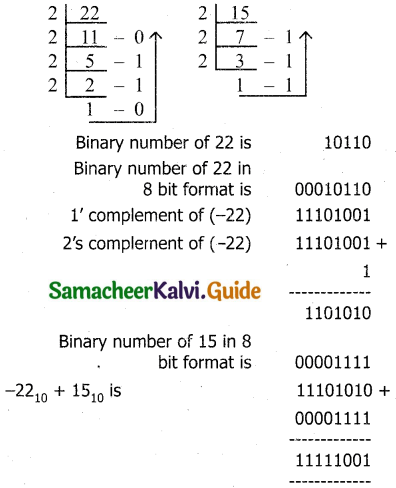Answer in 2’s complement form . 11111001 is 2’s complement of 7 which is the answer.Part – IV

Question 1.
a) Write the procedure to convert fractional
Decimal to Binary:
Conversion of fractional Decimal to Binary – The method of repeated multiplication by 2 has to be used to convert such kind of decimal fractions.

The steps involved in the method of repeated multiplication by 2:
Step 1 : Multiply the decimal fraction by 2 and note the integer part. The integer part is either O or 1.

Step 2: Discard the integer part of the previous product. Multiply the fractional part of the previous product by 2. Repeat Step 1 until the same fraction repeats or terminates (O).

Step 3 : The resulting integer part forms a sequence of Os and Is that become the binary equivalent of decimal fraction.

Step 4 : The final answer is to be written from first integer part obtained till the last integer part obtained.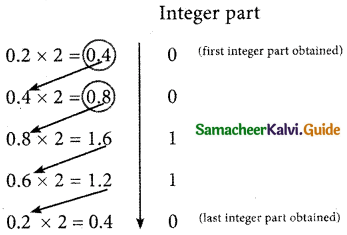Write the integer parts from top to bottom to obtain the equivalent fractional binary number.
Hence
(0.2)10 = (0.00110011…)2 = (0.00110011)2.

b) Convert (98.46)10 to Binary.
Convert (98.46)10 to Binary
Procedure : Conversion of integral part:Question 2.
Find 1’s Complement and 2’s Complement for the following Decimal number.
a) – 98
b) – 135
a) Conversion of (98)10 into binary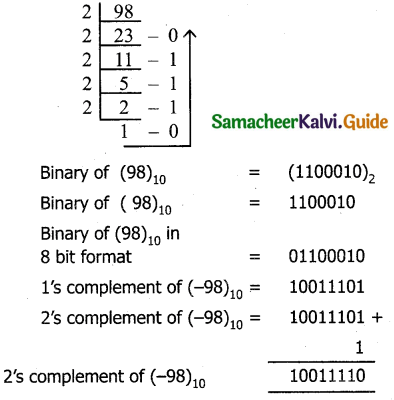b) Conversion of (135)10 into binary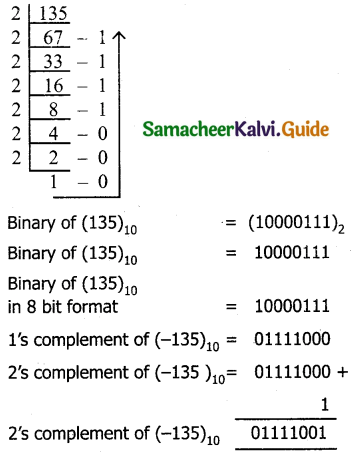Question 3.
Procedure :11010102 + 1011012 = 100101112

b) Subtract 11010112 – 1110102.
Subtract 11010112 – 1110102
Procedure :### 11th Computer Applications Guide Number Systems Additional Important Questions and Answers

Part – I

Question 1.
Computer handles data in the form of _________.
a) 1
b) 0
c) alphabets
d) both A and B
d) both A and B

Question 2.
Computer understand _________ language.
a) High level
b) Assembly
c) Machine
d) All the above
c) Machine

Question 3.
0 and 1 are called _________.
a) BIT
b) BYTE
c) NIBBLE
d) WORD
a) BITQuestion 4.
_________ is the basic unit of data in computer.
a) BIT
b) BYTE
c) NIBBLE
d) WORD
a) BIT

Question 5.
A _________ is the short form of Binary digit.
a) BIT
b) BYTE
c) NIBBLE
d) WORD
a) BIT

Question 6.
Binary digit means _________.
a) 0
b) 1
c) either 0 or 1
d) None of these
c) either 0 or 1

Question 7.
A _________ is a collection of 4 bits.
a) BIT
b) BYTE
c) NIBBLE
d) WORD
c) NIBBLE

Question 8.
A collection of 8 bits is called _________.
a) BIT
b) BYTE
C) NIBBLE
d) WORD
b) BYTE

Question 9.
The memory size of the computer is measured in the unit called _________.
a) BIT
b) BYTE
c) NIBBLE
d) WORD
b) BYTE

Question 10.
_________ refers to the number of bits processed by a computer’s CPU.
a) Word length
b) Nibble
c) Word size
d) None of these
a) Word length

Question 11.
The word length of the present day computer is _________ bits.
a) 32
b) 64
c) 8
d) 32 or 64
d) 32 or 64

Question 12.
_________ is a valid word length of a computer.
a) 64
b) 32
c) 16
d) All the above
d) All the above

Question 13.
_________ is not a valid word length of a computer.
a) 8
b) 12
c) 4
d) both B and C
d) both B and C

Question 14.
1 KiloByte equals to _________ bytes.
a) 1024
b) 256
c) 1000
d) 128
a) 1024

Question 15.
1 MegaByte equals to _________ KiloBytes.
a) 1024
b) 256
c) 1000
d) 128
a) 1024

Question 16.
1024 MegaBytes equals to _________.
a) 1 GigaByte
b) 1 TeraByte
c) 1 YottaByte
d) None of these
a) 1 GigaByte

Question 17.
1024 GigaByte equals to _________.
a) 1 GigaByte
b) 1 TeraByte
c) 1 YottaByte
d) None of these
b) 1 TeraByte

Question 18.
1Kb equals to _________ bytes.
a) 210
b) 220
c) 230
d) 240
a) 210Question 19.
1 MB equals to _________ bytes.
a) 210
b) 220
c) 230
d) 240
b) 220

Question 20.
1 GB equals to _________ bytes.
a) 210
b) 220
c) 230
d) 240
c) 230

Question 21.
1 TB equals to _________ bytes.
a) 210
b) 220
c) 230
d) 240
d) 240

Question 22.
1 PetaByte(PB) equals to _________ bytes.
a) 250
b) 260
c) 270
d) 280
a) 250

Question 23.
1 ExaByte (1EB) equals to _________ bytes.
a) 250
b) 260
c) 270
d) 280
b) 260

Question 24.
1 ZettaByte (1ZB) equals to _________ bytes.
a) 250
b) 260
c) 270
d) 280
c) 270

Question 25.
1 YottaByte equals to _________ bytes.
a) 250
b) 260
c) 270
d) 280
d) 280

Question 26.
Computer memory is normally represented in terms of _________ bytes.
a) Kilo
b) Mega
c) Kilo or Mega
d) None of these
c) Kilo or Mega

Question 27.
_________ are used to represent characters in a text.
a) Bytes
b) Bits
c) Nibbles
d) None of these
a) Bytes

Question 28.
The most commonly used codig scheme to represent character set and number is __________.
a) BCD
b) ASCII
c) EBCDIC
d) All the above
b) ASCII

Question 29.
ASCII is a _________ bit ccde.
a) 8
b) 7
c) 16
d) 32
b) 7

Question 30.
The ASCII value for blank space is _________.
a) 43
b) 42
c) 32
d) 62
c) 32Question 31.
The ASCII value for Numeric 0(zero) is _________.
a) 48
b) 38
c) 128
d) 32
a) 48

Question 32.
The most commonly used numbering system in real life is ________ number system.
b) Octal
c) Binary
d) Decimal
d) Decimal

Question 33.
Each number system is uniquely identified by its ________.
a) base value
d) symbols

Question 34.
________ is the count of number of digits in each number system.
a) base
d) symbols

Question 35.
________ is the general idea behind positional numbering system.
a) base value
d) symbols

Question 36.
Identify the true statement from the following.
a) In the positional number system, each decimal digit is weighted relative to its position in the number.
b) A numbering system is a way of representing numbers.
c) The speed of a computer depends on the number of bits it can process at once.
d) All the above
d) All the above

Question 37.
The left most bit in the binary number is called as the ________.
a) MSB
b) LSB
c) FSB
d) None of these
a) MSB

Question 38.
The right most bit in the binary number is called as the ________.
a) MSB
b) LSB
c) FSB
d) None of these
b) LSB

Question 39.
Each octal digit has its own positional value or weight as a power of ________.
a) 8
b) 16
c) 4
d) 10
a) 8

Question 40.
________ numbers are used as a shorthand form of binary sequence.
b) Octal
c) Decimal
d) None of these

Question 41.
________ system is used to represent data in a more compact manner.
b) Octal
c) Decimal
d) None of these

Question 42.
In hexadecimal number system letter ‘E’ represents ________.
a) 12
b) 13
c) 14
d) 15
c) 14

Question 43.
How many methods are there to convert decimal number to binary number?
a) only one
b) 2
c) 3
d) many
b) 2Question 44.
________ is a method to convert decimal number to binary number.
a) Repeated division by 2
b) Sum of powers of 2
d) Either A or B
d) Either A or B

Question 45.
Computer can handle ________ numbers.
a) positive
b) negative
c) positive and negative
d) None of these
c) positive and negative

Question 46.
Computer can handle ________ numbers.
a) signed
b) unsigned
c) signed and unsigned
d) None of these
c) signed and unsigned

Question 47.
The simplest method to represent negative binary numbers is called ________ method.
a) 1’s complement
b) 2’s complement
c) signed magnitude
d) All the above
c) signed magnitude

Question 48.
In signed magnitude method, the left most bit is called ________ bit.
a) sign
b) parity
c) sign or parity
d) None of these
c) sign or parity

Question 49.
How many ways a number can be represented in computers?
a) 3
b) 2
c) only one
d) None of these
a) 3

Question 50.
The numbers are represented in computers in ________ method.
a) Signed magnitude representation
b) 1’s complement
c) 2’s complement
d) All the above
d) All the above

Question 51.
If the number has ________ sign, it will be considered as positive in signed magnitute representation.
a) +
b) no
c) + or ++
d) A or B
d) A or B

Question 52.
If the number has ________ sign , it will be considered as negative in signed magnitute representation.
a) +
b) no
c) –
d) A or B
c) –

Question 53.
1’s complement of (00011000)2 is ________.
a) 11100111
b) 00011001
c) 00000011
d) None of these
a) 11100111

Question 54.
2’s complement of (0001i000)2 is ________.
a) 11100111
b) 00011001
c) 11101000
d) None of these
c) 11101000Question 55.
The binary numbers permits ________ computation.
b) multiplication
c) division
d) all the above
d) all the above

Question 56.
When two binary numbers are added ________ will be the output.
a) sum
b) carry
c) sum and carry
d) None of these
c) sum and carry

Question 57.
When subtracting 1 from 0, borrow 1 from the next ________.
a) LSB
b) MSB
c) either A or B
d) None of these
b) MSB

Question 58.
All the characters in the character set are denoted through ________ only.
a) numbers
b) alpha numeric
c) alphabet
d) None of these
a) numbers

Question 59.
________ is the character encoding system.
a) BCD and ISCII
b) EBCDIC
c) ASCII and Unicode
d) All the above
d) All the above

Question 60.
BCD stands for ________.
a) Binary Coded Decimal
b) Binary Character Decoding
c) Basic Coded Decimal
d) Bit Coded Decimal
a) Binary Coded Decimal

Question 61.
EBCDIC stands for ________.
a) Extensive Binary Coded Decimal Interchange Code
b) Extended Binary Coded Decimal Interchange Code
c) Extended Binary Coded Digit Interchange Code
d) Extended Bit Coded Decimal Interchange Code.
b) Extended Binary Coded Decimal Interchange Code

Question 62.
ASCII stands for ________.
a) Arithmetic Standard Code for Information Interchange
b) American Structured Code for Information Interchange
c) American Standard Code for Information Interchange
d) American Standard Code for Instant Interchange
c) American Standard Code for Information Interchange

Question 63.
ISCII stands for ________.
a) International Standard Code for Information Interchange
b) Indian Structured Code for Information Interchange
c) India’s Standard Code for Information Interchange
d) Indian Standard Code for Information Interchange
d) Indian Standard Code for Information Interchange

Question 64.
BCD is ________ bit code.
a) 6
b) 7
c) 8
d) None of these
a) 6

Question 65.
EBCDIC is ________ bit code.
a) 6
b) 7
c) 8
d) None of these bit code
c) 8Question 66.
ASCII is ___________ bit code.
a) 6
b) 7
c) 8
d) None of these
b) 7

Question 67.
Unicode is _________ bit code.
a) 16
b) 7
c) 8
d) None of these
a) 16

Question 68.
ISCII is ________ bit code.
a) 16
b) 7
c) 8
d) None of these
c) 8

Question 69.
________ coding system is formulated by IBM.
a) BCD
b) EBCDIC
c) ISCII
d) None of these
b) EBCDIC

Question 70.
IBM stands for ________.
b) International Basic Machine

Question 71.
________ is the system of handling the characters of Indian local languages.
a) ASCII
b) Unicode
c) BCD
d) ISCII
d) ISCII

Question 72.
ISCII system is formulated fay the ________ in India.
a) Department of Electronics
b) Department of Electricity
c) Department of E-commerce
d) Department of Economics
a) Department of Electronics

Question 73.
BCO system can handle ________ characters.
a) 64
b) 128
c) 256
d) 65536
a) 64

Question 74.
EBCDIC system can handle characters.
a) 64
b) 128
c) 256
d) None of these
c) 256

Question 75.
ASCII system can handle ________ characters.
a) 64
b) 128
c) 256
d) None of these
c) 256

Question 76.
Unicode system can handle ________ characters.
a) 64
b) 128
c) 256
d) 65536
d) 65536

Question 77.
ISCII system can handle ________ characters.
a) 64
b) 128
c) 256
d) 65535
c) 256

Question 78.
__________ language characters are not represented by ASCII.
a) Tamil
b) Malayalam
d) All the above
d) All the aboveQuestion 79.
Tamil, Malayalam, Telugu and kannda languages characters are represented by ________ code.
a) ASCII
b) Unicode
c) BCD
d) ISCII
b) Unicode

Question 80.
________ scheme is denoted by heaxdecimal numbers.
a) ASCII
b) Unicode
c) BCD
d) ISCII
b) Unicode

Question 81.
ISCII code was formulated in the year ________.
a) 1986 – 88
b) 1984 – 86
c) 1988
d) 1987
a) 1986 – 88

Question 82.
________ coding system is integrated with Unicode.
a) ASCII
b) EBCDIC
c) BCD
d) ISCII
d) ISCII

Question 83.
________ was generated to handle all the coding system of Universal languages.
a) ASCII
b) Unicode
c) BCD
d) ISCII
b) Unicode

Question 84.
The popular coding scheme after ASCII is ________.
a) EBCDIC
b) Unicode
c) BCD
d) ISCII
b) Unicode

Question 85.
BCD system is bit encoding system.
a) 28
b) 216
c) 26
d) 24
c) 26

Question 86.
EBCDIC svstem is ________ bit encoding system.
a) 28
b) 216
c) 26
d) 27
a) 28

Question 87.
ASCII system is ________ bit encoding system.
a) 28
b) 216
c) 26
d) 27
d) 27

Question 88.
Unicode system is ________ bit encoding system.
a) 28
b) 216
c) 26
d) 27
b) 216

Question 89.
ISCII system is ________ bit encoding system.
a) 28
b) 216
c) 26
d) 27
a) 28Question 90.
The input code in ASCII can be converted into ________ system.
a) EBCDIC
b) Unicode
c) BCD
d) ISCII
a) EBCDIC

Question 91.
What is ASCII value for ‘A’ in decimal number,
a) 97
b) 65
c) 98
d) 32
b) 65

Question 92.
What is ASCII value for ‘A’ in binary number.
a) 01100001
b) 01000001
c) 01100010
d) 00100000
b) 01000001

Question 93.
What is ASCII value for ‘A’ in octal number.
a) 141
b) 101
c) 142
d) 40
b) 101

Question 94.
What is ASCII value for ‘A’ in hexadeicmal number.
a) 61
b) 41
c) 62
d) 20
b) 41

Question 95.
Find the false statement in the following.
a) Computers can handle positive and negative numbers.
b) MSB is called as sign bit
c) LSB is called as parity bit
d) All the above
c) LSB is called as parity bit

Question 96.
Match the following.
a) 78 – (1) Binary number
b) 1111 – (2) Octal number
c) CAFE – (3) Decimal number
d) 71 – (4) Hexadecimal number
a) 3, 1, 4, 2
b) 4, 3, 2, 1
c) 1,3, 2,4
d) 3, 1,2,4
a) 3, 1, 4, 2

Question 97.
In signed magnitude representation, ________ in the sign bit represents negative number.
a) 0
b) 1
c) No symbol
d) None of these
b) 1

Question 98.
In signed magnitude representation, ________ in the sign bit represents positive number.
a) 0
b) 1
c) No symbol
d) None of these
a) 0

Question 99.
The term data comes from the word ________.
a) datum
b) date
c) fact
d) None of these
a) datumPart – II

Question 1.
Define data.
Data is an unprocessed collection of facts given as input to the computer. In a program, the values assigned to the variables are called data.

Question 2.
Define information.
Information is a processed facts and obtained from the computer as output. It convey meaning.

Question 3.
What you mean by nibble?
A nibble is a collection of 4 bits Binary digits.

Question 4.
Define Bit and Byte.

• Bit : A bit is the short form of Binary digit which can be ‘0’ or ‘1’. It is the basic unit of data in computers.
• Byte : A collection of 8 bits is called Byte. It is the basic unit of measuring the memory size in the computer.

Question 5.
What do you mean by word length?
Word length refers to the number of bits processed by a Computer’s CPU. For example, a word length can have 8 bits, 16 bits, 32 bits and 64 bits.

Question 6.
What are the different types of coding schemes to represent character set?
The different coding schemes are

1. BCD – Binary Coded Decimal
2. EBCDIC – Extended Binary Coded Decimal Interchange Code
3. ASCII – American Standard Code for Information Interchange
4. Unicode
5. ISCII – Indian Standard Code for Information Interchange.

Question 7.
Write note on number system.
A numbering system is a way of representing numbers. The most commonly used numbering system in real life is Decimal number system. Other number systems are Binary, Octal, and Hexadecimal Number System.Question 8.
What does base or radix mean?
Radix or base is the count of number of digits in each number system. Radix or base is the general idea behind positional numbering system.

Question 9.
Why the computers are working with different speed?
The speed of a computer depends on the number of bits it can process at once. For example, a 64- bit computer can process 64-bit numbers in one operation, while a 32-bit computer break 64- bit numbers down into smaller pieces, making it slower.

Question 10.
Write note on decimal number system.
It consists of 0, 1, 2, 3, 4, 5, 6, 7, 8, 9 (10 digits). It is the oldest and most popular number system used in our day to day life. In the positional number system, each decimal digit is weighted relative to its position in the number. Its base or radix is 10.

Question 11.
Octal number system uses digits 0, 1, 2, 3, 4, 5, 6 and 7 (8 digits). Each octal digit has its own positional value or weight as a power of 8. Its base or radix is 8.

Question 12.
How will you convert decimal to hexadecimal?
To convert Decimal to Hexadecimal, “Repeated division by 16” method can be used) In this method, we have to divide the given number by 16.

Example : Convert (31)10 into its equivalent hexadecimal number.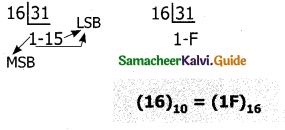Question 13.
Give the procedure to Octal to Binary.
Procedure: For each octal digit in the given number write its 3 digits binary equivalent using positional notation.

Example:
Convert (6213)8 to equivalent Binary number.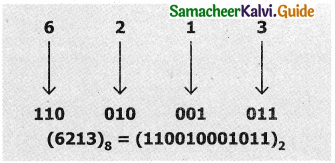Question 14.
How will you convert Hexadecimal to Binary?
Procedure : Write 4 bits Binary equivalent for each Hexadecimal digit for the given number using positional notation method.

Example:
Convert (8BC)16 into equivalent Binary number.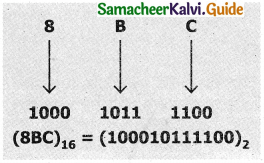Question 15.
Write short note on Binary Coded Decimal (BCD).
This is 26 bit encoding system. This can handle 26 = 64 characters only. This encoding system is not in the practice right now.

Question 16.
Write note on EBCDIC encoding system.
Extended Binary Coded Decimal Interchange Code (EBCDIC) is similar to ASCII Code with 8 bit representation. This coding system is formulated by International Business Machine (IBM). The coding system can handle 256 characters. The input code in ASCII can be converted to EBCDIC system and vice – versa.Question 17.
Write note on ISCXI encoding system.
ISCII is the system of handling the character of Indian local languages. This as a 8-bit coding system. Therefore it can handle 256 (28) characters. This system is formulated by the department of Electronics in India in the year 1986-88 and recognized by Bureau of Indian Standards (BIS). Now this coding system is integrated with Unicode.

Part – III

Question 1.
There are only two digits in the Binary system, namely, 0 and 1. The numbers in the binary system are represented to the base 2 and the positional multipliers are the powers of 2.

The left most bit in the binary number is called as the Most Significant Bit (MSB) and it has the largest positional weight. The right most bit is the Least Significant Bit (LSB) and has the smallest positional weight.Question 2.
A hexadecimal number is represented using base 16. Hexadecimal or Hex numbers are used as a shorthand form of binary sequence. This system is used to represent data in a more compact manner. Since 16 symbols are used, 0 to F, the notation is called hexadecimal.

The first 10 symbols are the same as in the decimal system, 0 to 9 and the remaining 6 symbols are taken from the first 6 letters of the alphabet sequence, A to F, where A represents 10, B is 11, C is 12, D is 13, E is 14 and F is 15.

Question 3.
Give the procedure to convert decimal to octal.
To convert Decimal to Octal, “Repeated Division by 8” method can be used) In this method, we have to divide the given number by 8.

Example:
Convert (65)10 into its equivalent Octal number.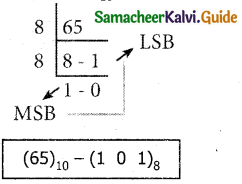Question 4.
Give the procedure to convert Octal to Decimal
To convert Octal to Decimal, we can use positional notation method:

1. Write down the Octal digits and list the powers of 8 from right to left (Positional Notation).
2. For each positional notation of the digit write the equivalent weight.
3. Multiply each digit with its corresponding weight.

Example:
Convert (1265)8 to equivalent Decimal number.(1265)8 = 512 x 1 + 64 x 2 + 8 x 6 + 1 x 5 = 512 + 128 + 48 + 5
(1265)8 = (693)10

Question 5.
How will you convert Hexadecimal to Decimal?
To convert Hexadecimal to Decimal we can use positional notation method:

1. Write down the Hexadecimal digits and list the powers of 16 from right to left (Positional Notation)
2. For each positional notation written for the digit, now write the equivalent weight.
3. Multiply each digit with its corresponding weight
4. Add all the values to get one final value.

Example:
Convert (25F)16 into its equivalent Decimal number.(25F)16 = 2 x 256 + 5 x 16 + 15 x 1 = 512 + 80 + 15
(25F)16 = (607)10Question 6.
Write about binary representation for signed numbers.
Computers can handle both positive (unsigned) and negative (signed) numbers. The simplest method to represent negative binary numbers is called Signed Magnitude. In signed magnitude method, the left most bit is Most Significant Bit (MSB), is called sign bit or parity bit.

The numbers are represented in computers in different ways:

1. Signed Magnitude representation
2. 1’s Complement
3. 2’s Complement

Question 7.
Explain ASCII code in detail.
This is the most popular encoding system recognized by United States. Most of the computers use this system. Remember this encoding system can handle English characters only. This can handle 27 bit which means 128 characters.

In this system, each character has individual number .The new edition ASCII -8, has 28 bits and can handle 256 characters are represented from 0 to 255 unique numbers.

The ASCII code equivalent to the uppercase letter ‘A’ is 65. The binary representation of ASCII (7 bit) value is 1000001. Also 01000001 in ASCII-8 bit.

Question 8.
Explain Unicode in detail.
This coding system is used in most of the modern computers. The popular coding scheme after ASCII is Unicode. ASCII can represent only 256 characters. Therefore English and European Languages alone can be handled by ASCII.

Particularly there was a situation, when the languages like Tamil, Malayalam, Kannada and Telugu could not be represented by ASCII.

Hence, the Unicode was generated to handle all the coding system of Universal languages. This is 16 bit code and can handle 65536 characters. Unicode scheme is denoted by hexadecimal numbers.

PART – IV

Book Evaluation

Question 1.
Explain decimal to binary conversion using Repeated Division by 2 method.
To convert Decimal to Binary “Repeated Division by 2” method can be used. Any Decimal number divided by 2 will leave a remainder of 0 or 1. Repeated division by 2 will leave a sequence of 0s and Is that become the binary equivalent of the decimal number.

Suppose it is required to convert the decimal number N into binary form, dividing N by 2 in the decimal system, we will obtain a quotient N1 and a remainder R1, where R1 can have a value of either 0 or 1. The process is repeated until the quotient becomes 0 or 1. When the quotient is ‘O’ or ‘1’, it is the final remainder value. Write the final answer starting from final remainder value obtained to the first remainder value obtained.

Example:
Convert (65)10 into its equivalent binary number.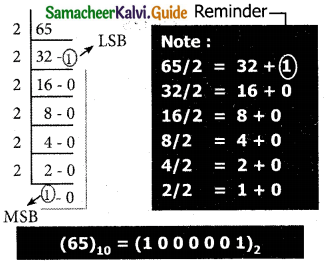Question 2.
Explain decimal to binary conversion using Sum of powers of 2 method.
A decimal number can be converted into a binary number by adding up the powers of 2 and then adding bits as needed to obtain the total value of the number.

a) Find the largest power of 2 that is smaller than or equal to 65.
6510 > 6410

b) Set the 64’s bit to 1 and subtract 64 from the original number
65 – 64 = 1

c) 32 is greater than the remaining total.
Therefore, set the 32’s bit to 0.

d) 16 is greater than the remaining total.
Therefore, set the 16’s bit to 0.

e) 8 is greater than the remaining total.
Therefore, set the 8’s bit to 0.

f) 4 is greater than the remaining total.
Therefore, set the 4’s bit to 0.

g) 2 is greater than the remaining total.
Therefore, set the 2’s bit to 0.

h) As the remaining value is equivalent to l’s bit, set it to 1.
1 – 1 = 0
Conversion is complete 6510 = (1000001)2

Example:
The conversion steps can be given as follows:
Given Number : 65
Equivalent or value less than power of 2 is : 64
(1) 65 – 64 = 1
(2) 1 – 1 = 0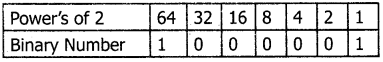6510 = (1000001)2.Question 3.
Explain the procedure to convert fractional decimal to Binary.
The method of repeated multiplication by 2 has to be used to convert such kind of decimal fractions. The steps involved in the method of repeated multiplication by 2:

Step 1: Multiply the decimal fraction by 2 and note the integer part. The integer part is either 0 or 1.

Step 2: Discard the integer part of the previous product. Multiply the fractional part of the previous product by 2. Repeat Step 1 until the same fraction repeats or terminates (0).

Step 3: The resulting integer part forms a sequence of Os and Is that become the binary equivalent of decimal fraction.

Step 4: The final answer is to be written from first integer part obtained till the last integer part obtained.

Integer partWrite the integer parts from top to bottom to obtain the equivalent fractional binary number. Hence (0.2)10 = (0.00110011…)2 = 0.00110011)2

Question 4.
How will you convert Binary to Decimal?
To convert Binary to Decimal we can use positional notation method.

• Step 1: Write down the Binary digits and list the powers of 2 from right to left (Positional Notation)
• Step 2: For each positional notation written for the digit, now write the equivalent weight.
• Step 3: Multiply each digit with its corresponding weight
• Step 4: Add all the values.

Example:
Convert (111011)2 into its equivalent decimal number.32 + 16 + 8 + 0 + 2 + 1 = (59)10
(111011)2 = (59)10

Question 5.
How will you convert Binary to Octal?

• Step 1: Group the given binary number into 3 bits from right to left.
• Step 2: You can add preceding 0 to make a group of 3 bits if the left most group has less than 3 bits.
• Step 3: Convert equivalent octal value using “2’s power positional weight method”

Example:
Convert (11010110)2 into octal equivalent number

Step 1: Group the given number into 3 bits from right to left.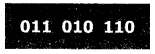The left most groups have less than 3 bits, so 0 is added to its left to make a group of 3 bits.

Step 2: Find Octal equivalent of each group.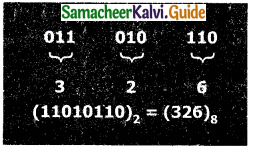Question 6.
Give the procedure to convert Binary to Hexadecimal.

• Step 1: Group the given number into 4 bits from right to left.
• Step 2: You can add preceding 0’s to make a group of 4 bits if the left most group has less than 4 bits.
• Step 3: Convert equivalent Hexadecimal value using “2’s power positional weight method”.

Example

Step 1: Group the given number into 4 bits from right to left.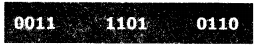0’s are added to the left most group to make it a group of 4 bits.Question 7.
Give the procedure to convert fractional Binary to Decimal equivalent.
The steps to convert fractional Binary number to its Decimal equivalent:
Step 1 : Convert integral part of Binary to Decimal equivalent using positional notation method.

Step 2 : To convert the fractional part of binary to its decimal equivalent.

• Write down the Binary digits in the fractional part.
• For all the digits write powers of 2 from left to right starting from 2-1, 2-2, 2-3 ….. 2-n, now write the equivalent weight.
• Multiply each digit with its corresponding weight.
• Add all the values which you obtained in Step 2.3.

Step 3 : To get final answer write the integral part (after conversion), followed by a decimal point(.) and the answer arrived at Step 2.4

Example:
Convert the given Binary number (11.011)2 into its decimal equivalent Integer part (11)2 = 3Question 8.
Explain the method of represent signed binary number in Signed Magnitude representation.
The value of the whole numbers can be determined by the sign used before it. If the number has ‘+’ sign or no sign it will be considered as positive. If the number has sign it will be considered as negative.

Example:

1. + 43 or 43 is a positive number
2. – 43 is a negative number

In signed binary representation, the left most bit is considered as sign bit. If this bit is 0, it is a positive number and if it 1, it is a negative number. Therefore a signed binary number has 8 bits, only 7 bits used for storing values (magnitude) and the 1 bit is used for sign.

1. +43 is represented in memory as follows:2. -43 can be represented in memory as follows.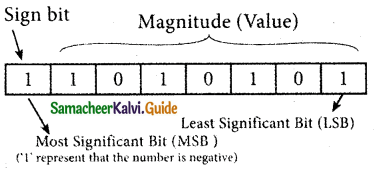Question 9.
Explain the method of represent signed binary number in l’s complement representation.
This is an easier approach to represent signed numbers. This is for negative numbers only i.e. the number whose MSB is 1.
The steps to be followed to find l’s complement of a number:

• Step 1 : Convert given Decimal number into Binary
• Step 2 : Check if the binary number contains 8 bits, if less add 0 at the left most bit, to make it as 8 bits.
• Step 3 : Invert all bits (i.e. Change 1 as 0 and 0 as 1)
Example: Find l’s complement for (-24)10Question 10.
Explain the method of represent signed binary number in 2’s complement representation.
The 2’s-complement method for negative number is as follows:
a) Invert all the bits in the binary sequence (i.e., change every 0 to 1 and every 1 to 0 i.e., 1’s complement)

b) Add 1 to the result to the Least Significant Bit (LSB).
Example:
2’s Complement represent of (-24)10Question 11.
Explain binary addition with suitable example.
The following table is useful when adding two binary numbers.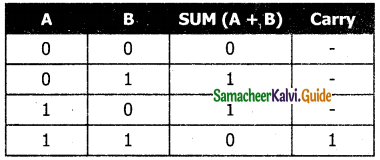In 1 + 1 = 10, is considered as sum 0 and the 1 as carry bit. This carry bit is added with the previous position of the bit pattern.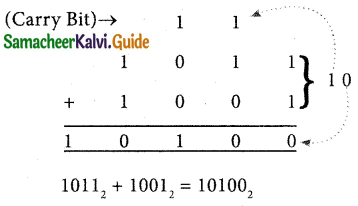Step 1 : Convert 23 and 12 into binary fromStep 2: Binary addition of 23 and 12:Question 11.
Explain binary subtraction with suitable example.
The table for Binary Subtraction is as follows: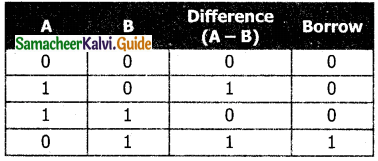When subtracting 1 from 0, borrow 1 from the next Most Significant Bit, when borrowing from the next Most Significant Bit, if it is 1, replace it with 0. If the next Most Significant Bit is 0, you must borrow from a more significant bit that contains 1 and replace it with 0 and 0s upto that point become Is.

Example : Subtract 10010102 – 101002.Example : Perform Binary addition for the following:
(-21)10 + (5)10

Step 1 :
Convert – 21 and 5 into binary formStep 2 :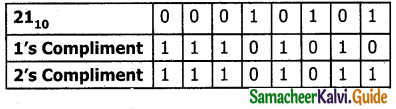Step 3 :
Binary Addition of – 21 and 5 :